# A Detailed Guide to Data Structures and Algorithms in JavaData structures are an integral part of a program. They are used to organize data in a specialized format that can be processed, stored, and retrieved quickly and effectively with the help of algorithms. In simpler terms, data structures are a means to simplify the handling and rendering of data.

It's even said that data structures and algorithms together make up the software program, consequently making it a quintessential concept that every programmer must master to land not just Java development jobs, others too.

In this tutorial, you will go through the concepts of data structures and algorithms in Java in detail. First, we will take you through the introduction to data structures and their classification. You'll also learn what algorithms are and how to measure their efficiency using time-complexity and space-complexity functions.

Without further ado, let’s get started!

## What is data structure?

Data + Structure. As the name implies, it is a way to arrange or organize data when writing a program. Depending on your requirement and project, it is extremely critical to choose the right data structure in Java. For instance, if you wish to store data sequentially, you might want to use the Array data structure -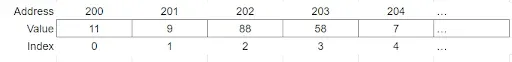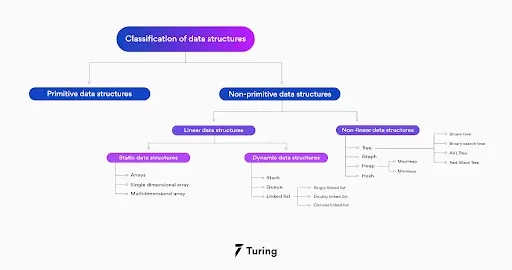## Classification of data structures

Now that we are familiar with data structures (DS), let’s move on to their classification. Data structures are divided into two categories.

Let’s have a look at each of these categories in detail -

### Primitive data structures

A primitive data structure consists of the int, char, float, double, and pointer data types that hold a single value.

### Non-primitive data structures

On the other hand, non-primitive data structures store data of more than one type. It can be categorized into two types:

#### Linear data structures

In linear data structures, data elements are positioned successively. Since the memory allocated as well are in sequential order, the implementation of such data structures is easy. It is further divided into - static and dynamic data structures.

#### Static data structures

In static data structures, memory is fixed in size, meaning the memory size is declared and fixed at the compile time which cannot be reallocated at a later point. A prominent example of such data structure is Array.

• Arrays*

Being the simplest data structure, the array allows storing data elements of the same type at contiguous memory locations. In Java, arrays are index-based which means that the elements can be accessed with the help of indices.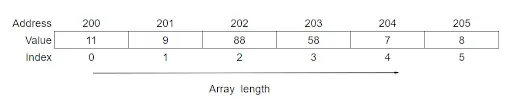##### Single dimensional array

Single dimensional arrays behave like a list of variables that can be accessed using an index in square brackets preceded by the name of that array. Syntax -

data_type[] array_name;

Or data_type array_name[];

Or data_type []array_name; //declaring array

array_name = new data_type[size]; // allocating memory to array

data_type array_name[] = {value1, value2, …valueN}; // initializing the array at declaration time

##### Multidimensional array

It is basically an array of arrays. Here the data elements are stored in a tabular format. Syntax -

data_type[1st dimension][2nd dimension][]..[Nth dimension] array_name = new data_type[size1][size2]….[sizeN]; //creating multidimensional arrays

arr_name[row_index][column_index]; accessing data element from 2-dimensional array

#### Dynamic data structures

A dynamic data structure or DDA is a structure that offers the flexibility to change its size during runtime, enabling the Java programmer to control exactly how much memory should be utilized. Examples of dynamic data structures are as follows:

##### Stack

As the name implies Stack data structure behaves like a real-world stack (e.g. pile of books). It follows the LIFO or Last-In-First-Out principle wherein push() and pop() operations are used to insert and delete the data. Additionally, it offers functionalities like:

• isEmpty() for verifying if the stack is empty or not
• isFull() for verifying if the stack is full or not
• peek() returns the element at the specific position
• count() returns the total number of elements present in the stack
• change() changes the element at the specified position
• display() prints all elements present in the stack
##### Queue

Queue data structure follows FIFO or First-In-First-Out principle wherein the elements are added from the end of the queue and deleted from the beginning of the queue. Few of the basic operations that this data structure allows are:

• enqueue() to add an element to the end of the queue
• dequeue() to delete an element from the front of the queue
• IsEmpty() to verify if the queue is empty
• IsFull() to verify if the queue is full
• peek() to get the value of the front of the queue without deleting it

Present in java.util package, the linked list is a sequence of data structures that are connected together via a link. Some of the operations that are performed on linked lists are:

• Traversal - accessing each node of the list
• Insertion - adding a new node to the list
• Deletion - deleting the existing node
• Search - finding a node from the list
• Sort - sorting the nodes in an order

It is divided into three categories:

• Singly linked list It is the most common form of a linked list wherein each element, also known as a node, contains a pointer linking to the next element in the sequence. So basically, each node contains two components - a value/data and a pointer pointing to the next node.

Here, the first node is called the head, and the last one is called the tail, which points to the null. In a singly linked list, nodes can be accessed linearly by traversing the list from head to tail.

• Doubly linked list Unlike the singly linked list, here, a node contains a pointer to the previous and the next node in the sequential list. A doubly linked list contains three components - a value/data, a pointer pointing to the next node (next pointer), and a pointer to the previous node (previous pointer).

• Circular linked list In a circular linked list, the last node of the list points to the first node of the list, and similarly first node of the list points to the last node of the list. Take a look at the below image for reference -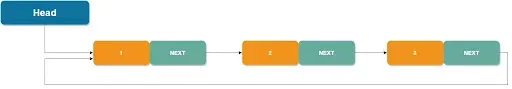#### Non-linear data structures

In this form of data structure, data elements are not organized, which means data elements are arranged in random order and do not involve a single level. When working with non-linear data structures, programmers cannot traverse all of its elements in a single run.

Let’s discuss some of the linear data structures:

##### Tree

It is a hierarchal data arranged in a tree-like structure consisting of nodes wherein each node stores a value. The tree data structure consists of a central/root node corresponding to various child/sub-nodes which are connected via edges.

• Binary tree It is a tree data structure that at most has 2 child nodes (names left and right nodes). It is implemented with the help of pointers.

• Binary search tree Also known as an ordered or sorted binary tree, a binary search tree is similar to a binary tree with the only difference being -

1. The left node must contain a smaller value than the parent node
2. The right node must contain a bigger value than the parent node
3. Both the right and left node must also be a binary search tree
• AVL Tree It is a balanced binary search tree where the difference between the heights of left and right subtrees cannot be less than or equal to 1. For instance, suppose TL and TR are two subtrees, then hL - hR <= 1. Here hL and hR are the height of the two subtrees.

• Red-Black Tree It is a self-balancing binary search tree where each node has an extra bit, represented with a color (either black or red). This is done to ensure that the tree remains balanced during the insertion and deletion of the nodes.

Even though this data structure is not as balanced as the AVL tree, it significantly reduces the rotations during insertion and deletion, making it a perfect choice for applications involving frequent insertions and deletions.

##### Graph

It is a pictorial representation of nodes (sometimes also referred to as vertices) connected via a link known as edges.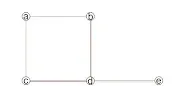The above graph consists of vertices or V = {a, b, c, d, e} and edges or E = {ab, ac, bd, cd, de}. Graph data structures are usually a representation of a network that is used to solve many real-life problems.

##### Heap

A Heap data structure is a complete binary tree. A complete binary tree is a binary tree where all nodes except the last nodes (known as the leaf node) are completely filled. All the nodes in a complete binary tree should be left-justified. Heap is divided into two categories:

1. MaxHeap: The root node's value should be greater than or equal to its child nodes. It can be represented as - A[Root(i)] >= A[i]
2. MinHeap: The value of the root node should be lesser than or equal to its child nodes. It can represented as - A[Root(i)] <= A[i]
##### Hash

Hash, also known as hash map, is a data structure that stores data in an associative manner. Here the stored data has a unique index value. These index values then can be utilized to access the stored data quickly.

## What is an algorithm?

Historically, algorithms were used as a tool for mathematical computation. In the present context, algorithms are linked with computer science, particularly with data structures. That’s the reason why we always discuss data structures and algorithms together.

In computer programming terms, an algorithm is a set of instructions that performs a specific task in a specific amount of time. For example - Here is set of instructions for An algorithm to add two numbers:

• Take two number inputs
• Add numbers using the + operator
• Display the result

### Qualities of a good Algorithm

• It receives at least one input and generates at least one output
• Input and output are precisely defined
• Each step of the algorithm is clear and precise
• It should be the most effective solution among many possible solutions to a problem.
• Computer code should not be included in an algorithm. Instead, the algorithm should be written in a way that allows it to be used in any of programming language
• It comes to an end, after certain number of steps

## How to use flowcharts and pseudocode to represent algorithms?

Do you know what the best way to represent an algorithm is? What's the best alternative to writing code before fully understanding its underlying algorithm?

Answers to these questions are Flowcharts and Pseudocode. So, let's look at how flowcharts and pseudocode can help us understand the algorithm simply and understandably.

### Using Flowcharts to represent algorithms

A flowchart is a visual representation of the control flow of an algorithm. It depicts statements that must be executed, decisions that must be made, logic flow (for iteration and other purposes), and terminals representing start and endpoints.

The figure below depicts the various symbols used to visualize algorithms in flowcharts.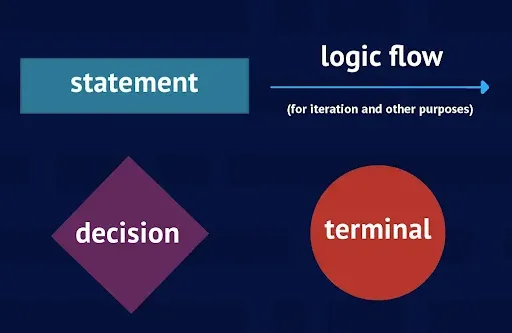### Using Pseudocode to represent algorithms

Pseudocode is a textual representation of an algorithm approximating the final source code. It is useful for quickly writing down the representation of an algorithm. There are no hard and fast rules for writing pseudocode as it does not have any syntax.

It can be replaced with flowcharts very quickly, when writing pseudocode, you should strive for consistency. Being consistent will make translating the pseudocode into actual source code much more accessible.

## Choosing the correct data structure and algorithm in Java

The data structures and algorithms in Java significantly impact two aspects of applications:

• Memory consumption (for data structures).
• CPU usage (for algorithms that interact with those data structures).

As a result, you should be especially careful about the data structures and algorithms to use in Java. These include applications used for big data and the internet of things.

When selecting a data structure or algorithm java, you will notice an inverse relationship between memory usage and CPU time. The less memory a data structure uses, the more CPU time, algorithms require to process the data items in the data structure. Furthermore, the more memory a data structure consumes, the less CPU time, algorithms will require to process the data items, resulting in faster algorithm results.

You should try to balance memory usage and CPU time as much as possible. This task can be simplified by analyzing algorithms to determine their efficiency.

### Measuring the efficiency of algorithms

Computer resources are limited and should be used well so we can say that various resources can be used to determine the efficiency of an algorithm. For maximum efficiency of the algorithm, you have to minimize the resource usage.

Time complexity and Space complexity are used for the efficiency of an algorithm, distilling these into complexity functions to abstract implementation and runtime environment details. The variance in an algorithm's time and space requirements based on the amount of input data is revealed by complexity functions:

• A time-complexity function quantifies an algorithm's time complexity or how long it takes to complete.
• A space-complexity function measures the space complexity of an algorithm, which is the amount of memory required by the algorithm to perform its task.

Both complexity functions are based on the input (n) size, which reflects the amount of input data. Let’s take an example to get a better understanding of it. Consider the following pseudocode for array printing:

DECLARE INTEGER i, x[] = [ 10, 15, -1, 32 ]

FOR i = 0 TO LENGTH(x) - 1

PRINT x[I]

NEXT I

END

### Time complexity

You can express the time complexity of this algorithm by specifying the time-complexity function t(n) = an+b, where a (a constant multiplier) represents the amount of time to complete one loop iteration, and b represents the algorithm's setup time.

### Space complexity:

The space complexity of an algorithm indicates how much extra memory is required to complete its task. No matter how large the array, a constant amount of extra memory (for code storage, stack space to store the return address when PRINT is called, and space for variable i's value) is required for printing.

The space complexity of the array-printing algorithm can be expressed using the space-complexity function s(n) = c, where c represents how much constant additional space is required. This value only represents overhead; it does not include data processing space. It does not include the array in this case.

## Conclusion

Data structures and algorithms (DSA) provide solutions through deeper insights into a standard problem. It also enables developers to find the efficiency of each of those solutions. After a thorough understanding of data structures and algorithms in Java, we can easily conclude that learning how to classify data structures, represent algorithms using flowcharts and pseudocode, and calculate their efficiency using time and space complexity functions is crucial. The bottom line is that DSA is very important to enter into software engineering.

## Frequently Asked Questions

Data structure is the arrangement of data in a program. The arrangement of data determines how a program works and how it will behave. Data structure is one of the most important concepts in programming. It determines the size and complexity of a program, the program’s speed, and the program’s ability to carry out instructions.

Data structures and algorithms are not language-specified and can be used for any programming language Java included.

Programming algorithms is the process of designing programs to carry out specific tasks. Algorithms are sets of instructions describing the steps required to carry out a particular task. An algorithm flowchart is a diagram that shows the sequence of steps in an algorithm. The shape of the flowchart depends on the task being performed.

Some commonly used data structures in Java are:

• Array
• Stack
• Queue
• Binary tree
• Binary search tree
• Heap
• Hashing
• Graph

An algorithm must have the following characteristics:

• Unambiguous
• Input
• Output
• Finiteness
• Feasibility
• Independent

Pseudocode is not a programming language and does not have any specific syntax.### Press

What's up with Turing? Get the latest news about us here.### Blog

Know more about remote work.
Checkout our blog here.### Contact

Have any questions?
We'd love to hear from you.

#### Hire and manage remote developers

Tell us the skills you need and we'll find the best developer for you in days, not weeks.